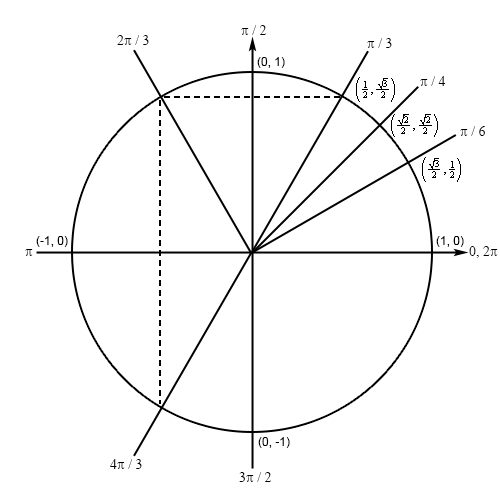Paul's Online Notes
Home / Calculus I / Review / Solving Trig Equations
Show Mobile Notice Show All Notes Hide All Notes
Mobile Notice
You appear to be on a device with a "narrow" screen width (i.e. you are probably on a mobile phone). Due to the nature of the mathematics on this site it is best views in landscape mode. If your device is not in landscape mode many of the equations will run off the side of your device (should be able to scroll to see them) and some of the menu items will be cut off due to the narrow screen width.

### Section 1.4 : Solving Trig Equations

12. Without using a calculator find the solution(s) to $$\displaystyle 15 = 17 + 4\cos \left( {\frac{y}{7}} \right)$$ that are in $$\left[ {10\pi ,15\pi } \right]$$.

Show All Steps Hide All Steps

Hint : Find all the solutions to the equation without regard to the given interval. The first step in this process is to isolate the cosine (with a coefficient of one) on one side of the equation.
Start Solution

Isolating the cosine (with a coefficient of one) on one side of the equation gives,

$\cos \left( {\frac{y}{7}} \right) = - \frac{1}{2}$
Hint : Use your knowledge of the unit circle to determine all the angles in the range $$\left[ {0,2\pi } \right]$$ for which cosine will have this value.
Show Step 2

Because we’re dealing with cosine in this problem and we know that the $$x$$-axis represents cosine on a unit circle we’re looking for angles that will have a $$x$$ coordinate of $$- \frac{1}{2}$$. This means that we’ll have angles in the second and third quadrant.

Because of the negative value we can’t just find the corresponding angle in the first quadrant and use that to find the second angle. However, we can still use the angles in the first quadrant to find the two angles that we need. Here is a unit circle for this situation.If we didn’t have the negative value then the angle would be $$\frac{\pi }{3}$$. Now, based on the symmetry in the unit circle, the terminal line for both of the angles will form an angle of $$\frac{\pi }{3}$$ with the negative $$x$$-axis. The angle in the second quadrant will then be $$\pi - \frac{\pi }{3} = \frac{{2\pi }}{3}$$ and the angle in the third quadrant will be $$\pi + \frac{\pi }{3} = \frac{{4\pi }}{3}$$.

Hint :Using the two angles above write down all the angles for which cosine will have this value and use these to write down all the solutions to the equation.
Show Step 3

From the discussion in the notes for this section we know that once we have these two angles we can get all possible angles by simply adding “$$+ \,2\pi n$$ for $$n = 0, \pm 1, \pm 2, \ldots$$” onto each of these.

This then means that we must have,

$\frac{y}{7} = \frac{{2\pi }}{3} + 2\pi n \hspace{0.5in} {\rm{OR }} \hspace{0.5in} \frac{y}{7} = \frac{{4\pi }}{3} + 2\pi n \hspace{0.5in} n = 0, \pm 1, \pm 2, \ldots$

Finally, to get all the solutions to the equation all we need to do is multiply both sides by 7.

$y = \frac{{14\pi }}{3} + 14\pi n \hspace{0.5in} {\rm{OR }} \hspace{0.5in} y = \frac{{28\pi }}{3} + 14\pi n \hspace{0.5in} n = 0, \pm 1, \pm 2, \ldots$
Hint : Now all we need to do is plug in values of $$n$$ to determine which solutions will actually fall in the given interval.
Show Step 4

Note that because at least some of the solutions will have a denominator of 3 it will probably be convenient to also have the interval written in terms of fractions with denominators of 3. Doing this will make it much easier to identify solutions that fall inside the interval so,

$\left[ {\frac{{30\pi }}{3},\frac{{45\pi }}{3}} \right]$

With the interval written in this form, if our potential solutions have a denominator of 3, all we need to do is compare numerators. As long as the numerators are between $$30\pi$$ and $$45\pi$$ we’ll know that the solution is in the interval.

Also, in order to quickly determine the solution for particular values of $$n$$ it will be much easier to have both fractions in the solutions have denominators of 3. So, the solutions, written in this form, are.

$y = \frac{{14\pi }}{3} + \frac{{42\pi n}}{3} \hspace{0.5in} {\rm{OR }} \hspace{0.5in} y = \frac{{28\pi }}{3} + \frac{{42\pi n}}{3} \hspace{0.5in} n = 0, \pm 1, \pm 2, \ldots$

Now let’s find all the solutions. First notice that, in this case, if we plug in negative values of $$n$$ we will get negative solutions and these will not be in the interval and so there is no reason to even try these. We can also see from a quick inspection that $$n = 0$$ will result in solutions that are not in the interval and so let’s start at $$n = 1$$ and see what we get.

$\begin{array}{llcl}{n = 1:} & {x = \require{cancel} \xcancel{{\frac{{56\pi }}{3}}} > \frac{{45\pi }}{3}\,} & {\,\,\,{\rm{OR}}\,\,\,} & {x = \xcancel{{\frac{{70\pi }}{3}}} > \frac{{45\pi }}{3}}\end{array}$

So, by plugging in $$n = 1$$ we get solutions that are already outside of the interval and increasing $$n$$ will simply mean adding another $$\frac{{42\pi }}{3}$$ onto these and so will remain outside of the given interval. We also noticed earlier than all other value of $$n$$ will result in solutions outside of the given interval.

What all this means is that while there are solutions to the equation none fall inside the given interval and so the official answer would then be no solutions in the given interval.